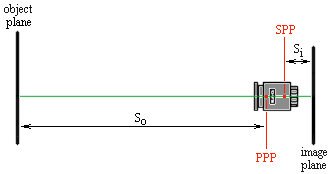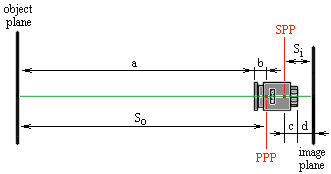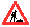# Focal Length Experiments

## or “My Macro Gets Wider as I Focus Closer?!?!”

Yes, it is possible for a prime lens to change its focal length. This happens if the lens design is such that during refocusing some optical elements change their position with respect to other optical elements. Examples of such designs are lenses with internal focusing (IF) and Pentax lenses with fixed rear extension elements (FREE). While these designs achieve various positive results, they typically lead to a decreased focal length when the lens is focused closer than infinity.

One of my favorite lenses is the Pentax SMC-A 100/2.8 Macro which has a FREE design. And since a macro lens is most often used at small focus distances, I want how wide it gets as I get close…

## Problem

1. Develop one or more methods for calculating the focal length of a lens.
2. Apply these methods to the SMC Pentax-A Macro 1:2.8 100 mm lens in order to find its focal length at various focusing distances, from infinity up to 1:1.

## Known

When a lens forms a focused image of an object, the following is true:

1) 1 / So + 1 / Si = 1 / f
2) M = Si / So

where:

So – distance between the object and the primary principal point (PPP) of the lens,
Si – distance between the secondary principal point (SPP) of the lens and the formed image,
f – lens focal length,
M – image magnification.

Figure 1 illustrates.Figure 1.

The positions of the PPP and SPP depend solely on the optical properties of the lens, and So and Si are generally unknown. For definitions of PPP and SPP see the lens principal points page.

## Solution, Part 1

To tackle the problem, we split So and Si into two sums:

3) So = a + b,
4) Si = c + d,

where the boundary between a and b is arbitrarily chosen to be the front-most part of the filter ring and between c and d the rear-most part of the lens mount. In this way the quantities b and c measure how deep the PPP and SPP are located inside the lens.

Figure 2 illustrates.Figure 2.

We then substitute (3) and (4) into (1) and (2):

5) 1 / (a + b) + 1 / (c + d) = 1 / f,
6) M = (c + d) / (a + b),

and armed with these expressions, we have a number of alternatives for finding f:

## Solution, Part 2

Now we apply our three methods in order to find out how the focal length of the Pentax SMC-A 100/2.8 Macro lens changes with refocusing.

We calculate f for the following points: M = 1:1; M = 1:1.2; M = 1:1.5; M = 1:2; M = 1:2.5; M = 1:3; M = 1:4; M = 1:5; M = 1:10; M = 1:25; So = infinity.

Figure 3 shows a plot of the results.A14 = 416 mm, C14 = 77 mm => f(1:4) = 84.85 mm,
A13 = 325 mm, C13 = 74 mm => f(1:3) = 83.67 mm,
A12 = 220 mm, C12 = 70 mm => f(1:2) = 74.75 mm,
A11 = 122.5 mm, C11 = 61 mm => f(1:1) = 61.50 mm.